# How to Divide Decimals

“Learning how to divide decimals doesn’t have to be complicated,  just try not to forget the point.”Author
Amber WatkinsPublished
November 7, 2023# How to Divide Decimals

“Learning how to divide decimals doesn’t have to be complicated,  just try not to forget the point.”Author
Amber WatkinsPublished
Nov 7, 2023# How to Divide Decimals

“Learning how to divide decimals doesn’t have to be complicated,  just try not to forget the point.”Author
Amber WatkinsPublished
Nov 7, 2023Key takeaways

• Dividing decimals requires a good understanding of basic long division steps. If students know how to do long division, they can easily learn how to divide decimals.

• The skill of dividing decimals can be applied to a variety of fifth-grade math problems, including problems that involve dividing decimals in the form of money.

When we get paid for our summer chores or want to buy our favorite gaming apps, we are using money in the form of decimals. What if we need to divide that money by the price of the items we buy, or by the number of days we work?

We would need to know how to divide decimals, and because it involves our money, we want to know how to do it right!

In this article, we will learn how to divide decimals by whole numbers and how to divide decimals by other decimals. Through this guided practice we will be prepared to apply this knowledge to classroom math problems and in real-world scenarios.

## Dividing Decimals Step by Step### Dividing Decimals by Whole NumbersWhen dividing decimals by whole numbers we follow the same steps as dividing whole numbers:

• Divide
• Multiply
• Subtract
• Bring down
• Repeat as necessary

However, the rule when dividing decimals by whole numbers is: When the dividend, the number you are dividing, has a decimal and you are dividing it by a whole number, there should also be a decimal in the answer.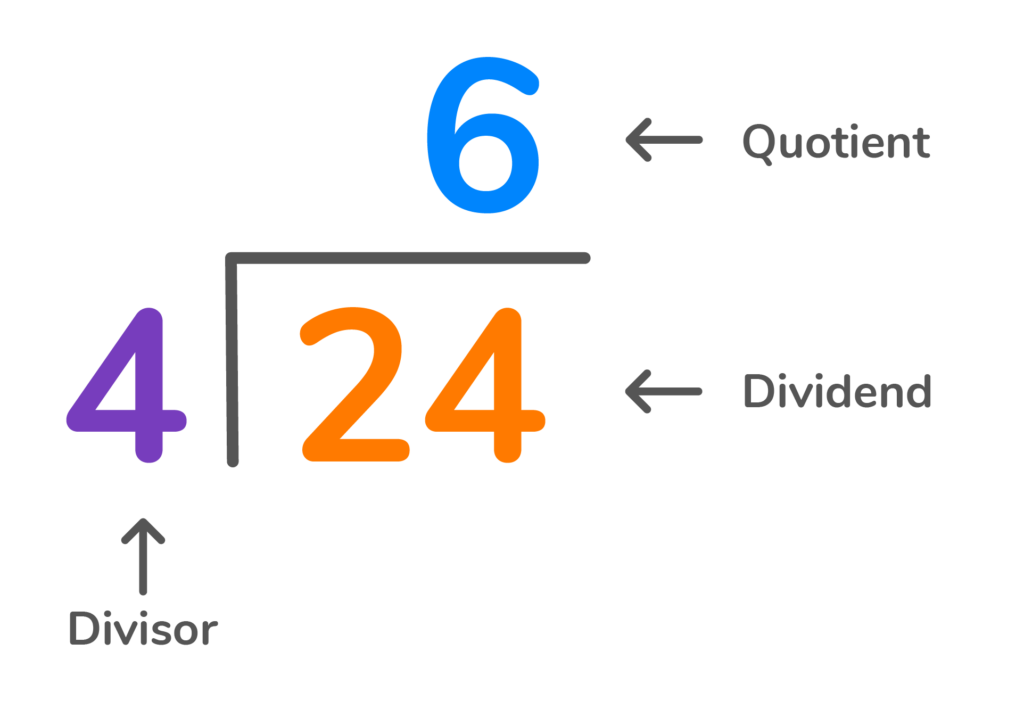The dividend is the number being divided.

The divisor is the number outside of the division bar, the number you are dividing by.

The quotient is the answer on top.

When completing long division with decimals, it is important to place the decimal point in the answer in the same place it appears in the dividend. An example of this is shown below.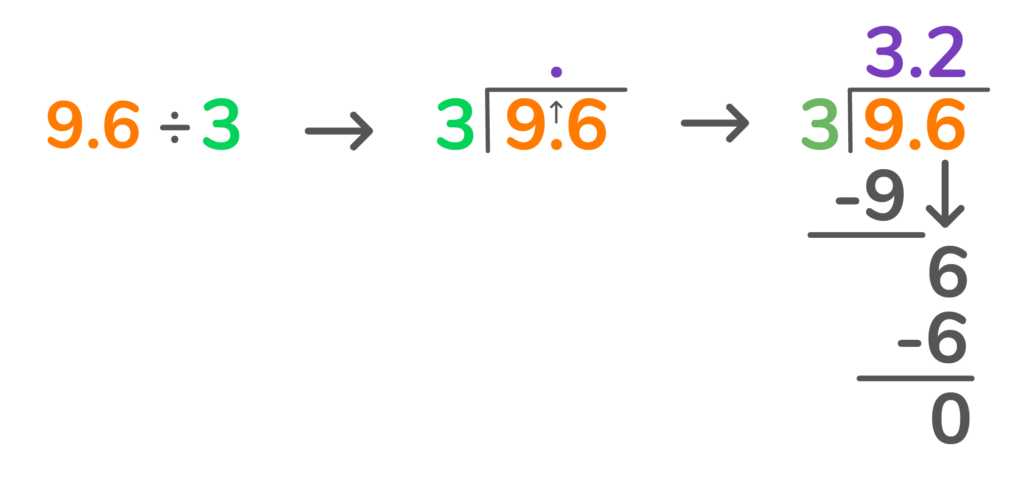It is strongly recommended to complete this step first in any long-division problem with decimals. That way, you never forget it! Remember, an answer of 35 is completely different than an answer of 3.5. So, don’t forget your decimal points!

Let’s practice: Where would you place the decimal in the answer to this long-division problem?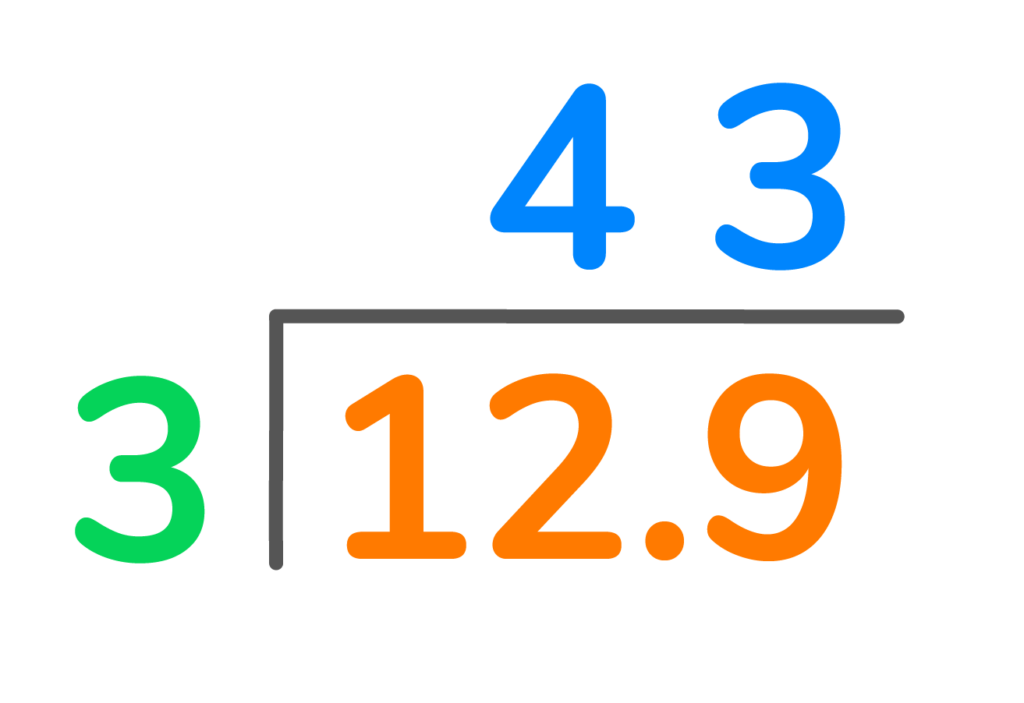If you guessed between the numbers 4 and 3  you are right!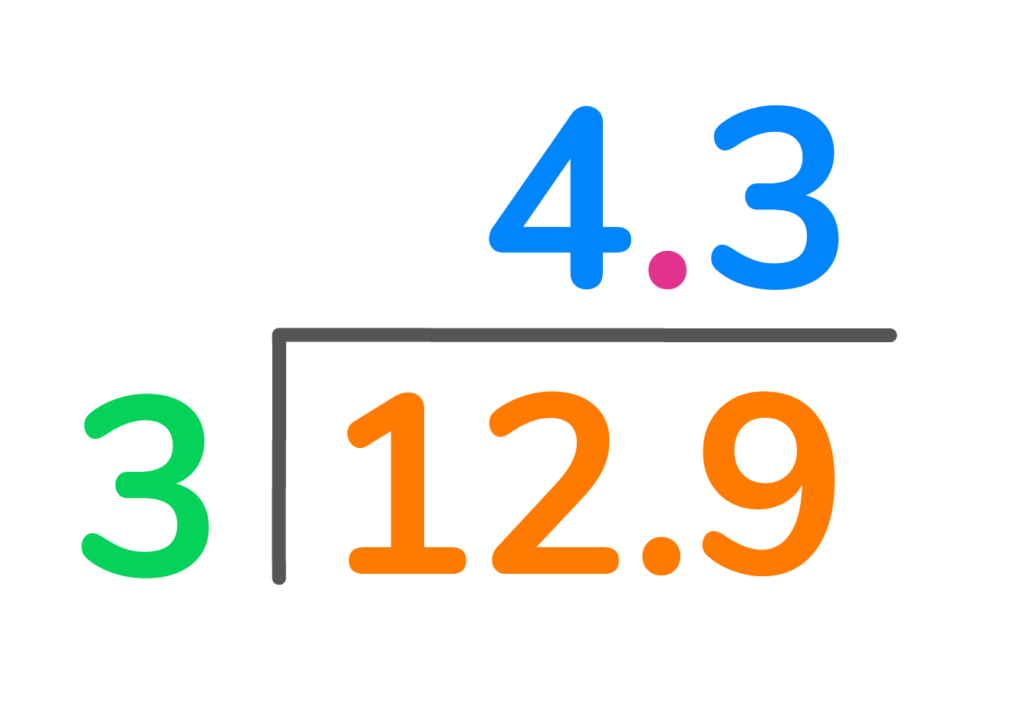## How Do You Divide Decimals Step by Step?

1. Dividing with decimals begins with placing the decimal point in the answer in the same place it is in the dividend.

2. Once the decimal point is in the quotient, or answer, you can begin the dividing process; divide, multiply, subtract, bring down, and repeat as necessary.

Would you like to see how this is done? Follow along to the decimal division problem below.

What is 4.524 divided by 2?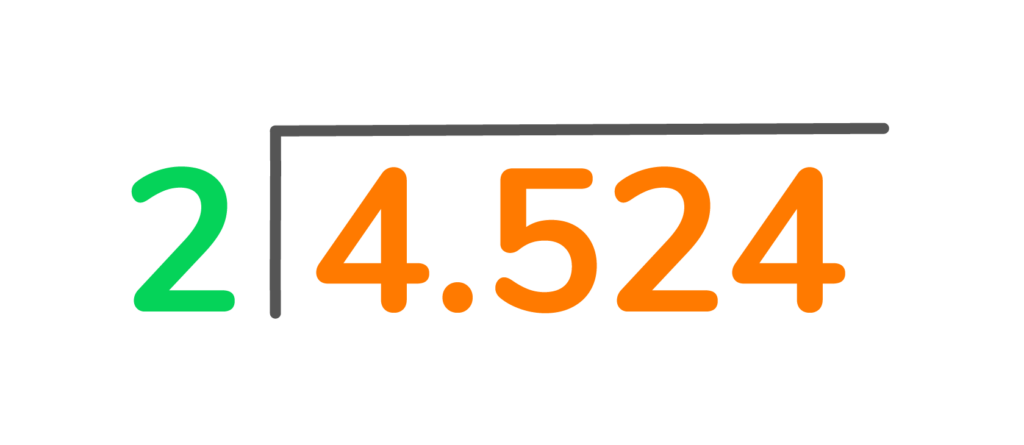Place the decimal above the division bar in the same place it is in the dividend.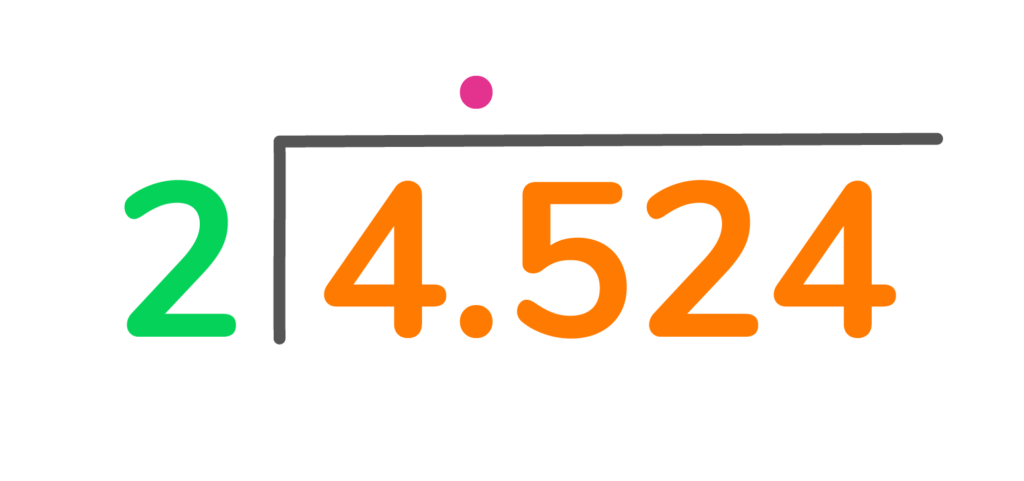Follow the basic long division steps: divide, multiply, subtract, bring down, and repeat as necessary.## Dividing Decimals by DecimalsDividing by decimals can be challenging. That is why when dividing decimals by decimals, the first step is to change the divisor into a whole number.

For example, if you are dividing 4.25 by .05, the divisor .05 is a decimal.

Dividing by a decimal will make the division problem more complicated. To make it easier, it is suggested to change the divisor into a whole number.

Let’s see how this is done. To change the decimal .05 to a whole number, you must move the decimal place over to the right two times.

The new divisor you will end up with is the whole number 5. It is easier to divide decimals by whole numbers. But there’s still more to do. Let’s review all of the steps when dividing decimals by decimals. Then let’s complete problems together.

## How to Divide Decimals by Other Decimals1. Change the divisor to a whole number by moving the decimal place over until there aren’t any decimal places left. Keep track of how many places you moved the decimal and how in which direction, to the left or right.

2. Move the decimal place of the dividend in the same direction and the same amount of times as you moved in the divisor.

3. Now follow the same rules as other long division problems: place the decimal in the dividend in the quotient, then divide, multiply, subtract, bring down, and repeat as many times as necessary.

Now, let’s practice together some division with decimals problems. Then you will have an opportunity to practice a few on your own.

## Dividing Decimals by Decimals Example ProblemWhat is 4.25 divided by .05?

Move the decimal place over two places to the right in the divisor, and two places to the right in the dividend.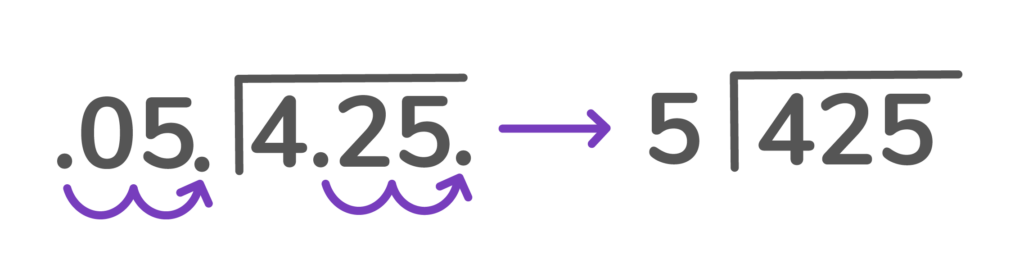Now follow the same steps: divide, multiply, subtract, bring down, and repeat as necessary.

## Practice problems

Click on the boxes below to see the answers!

• Set up the question as a long division problem
• Place the decimal point in the answer directly above the decimal in the dividend

Divide, multiply, subtract, bring down, and repeat as necessary

• Set up the question as a long division problem
• Place the decimal point in the answer directly above the decimal in the dividend

Divide, multiply, subtract, bring down, and repeat as necessary

• Set up the question as a long division problem
• Move the decimal one to the right in 1.5 to make it 15.
• Move the decimal one to the right in 7.5 to make 75.
• Now the new equation is 75 / 15 =

Divide, multiply, subtract, bring down, and repeat as necessaryYou divide decimals using the same steps as you would dividing whole numbers: divide, multiply, subtract, bring down, and repeat as necessary. The only difference is division of decimals begins with placing the decimal point in the quotient right above the decimal in the dividend.

You begin decimal division by placing the decimal point in the dividend in the quotient. Then follow the normal long division steps: divide, multiply, subtract, bring down, and repeat as necessary.

When dividing decimals by decimals, the divisor should be changed to a whole number by moving the decimal. Then you must change the dividend by moving the decimal the same amount of times in the same direction. Once this is complete, you can follow the normal long division steps: divide, multiply, subtract, bring down, and repeat if necessary.Lesson credits

Amber Watkins

Amber is an education specialist with a degree in Early Childhood Education. She has over 12 years of experience teaching and tutoring elementary through college level math. "Knowing that my work in math education makes such an impact leaves me with an indescribable feeling of pride and joy!"

Amber Watkins

Amber is an education specialist with a degree in Early Childhood Education. She has over 12 years of experience teaching and tutoring elementary through college level math. "Knowing that my work in math education makes such an impact leaves me with an indescribable feeling of pride and joy!"# Are you a parent, teacher or student?

Are you a parent or teacher?

## Hi there!

Book a chat with our team